### Population: P, P'

The maximal populationhas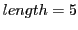over an alphabet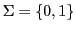. Thus we haveelements in the alphabet with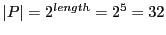.

The working set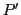shall be a subset ofwith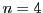many elements in. The real values of the elements ofwill be chosen by chance.

Using the scilab program GA_v4.sec15.2 we can call the function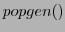to generate a working population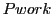with 4 many members, each member with the length=5:

``` -->n=4, l=5,[Pwork]=popgen(n,l)
n  =

4.
l  =

5.
Pwork  =

0.    1.    0.    0.    0.
0.    1.    0.    0.    1.
1.    0.    0.    1.    0.
1.    0.    0.    0.    0.
```

Gerd Doeben-Henisch 2013-01-14